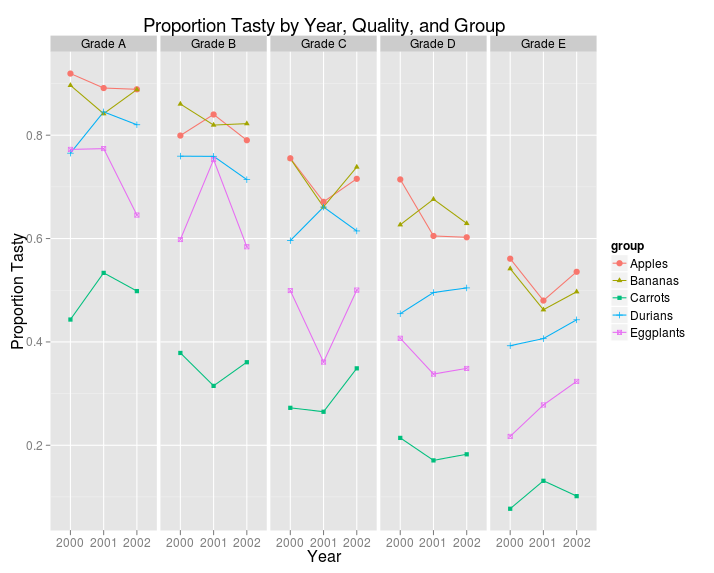# Jeromy Anglim's Blog: Psychology and Statistics

## Thursday, May 3, 2012

### How to plot three categorical variables and one continuous variable using ggplot2

This post shows how to produce a plot involving three categorical variables and one continuous variable using ggplot2 in R.

##### 1. Create Data

First, let's load ggplot2 and create some data to work with:

library(ggplot2)

set.seed(4444)
Data <- expand.grid(group=c("Apples", "Bananas", "Carrots", "Durians",
"Eggplants"),
year=c("2000", "2001", "2002"),
quality=c("Grade A", "Grade B", "Grade C", "Grade D",
"Grade E"))
Group.Weight <- data.frame(
group=c("Apples", "Bananas", "Carrots", "Durians", "Eggplants"),
group.weight=c(1,1,-1,0.5, 0))
Quality.Weight <- data.frame(
quality=c("Grade A", "Grade B", "Grade C", "Grade D", "Grade E"),
quality.weight = c(1,0.5,0,-0.5,-1))
Data <- merge(Data, Group.Weight)
Data <- merge(Data, Quality.Weight)
Data$score <- Data$group.weight + Data$quality.weight + rnorm(nrow(Data), 0, 0.2) Data$proportion.tasty <- exp(Data$score)/(1 + exp(Data$score))

##### 2. Produce Plot

And here's the code to produce the plot.

ggplot(data=Data,
aes(x=factor(year), y=proportion.tasty,
group=group,
shape=group,
color=group)) +
geom_line() +
geom_point() +
opts(title =
"Proportion Tasty by Year, Quality, and Group") +
scale_x_discrete("Year") +
scale_y_continuous("Proportion Tasty") +
facet_grid(.~quality )


And here's what it looks like:#### 1 comment:

1.Hi, I’m trying to plot a barplot with 3 categoricals by one continuous variable. It’s easy to do in an excel or google spreadsheet, but the ggplot2 code is a bit hard, but I think might be similar to what you've done. What I need is the y axis data (V) grouped by two time periods (0, 60) for one categorical group (NW, OB) and another (M,F) so you have four bars in two groups (by gender and by body weight). I’m using the mean values of V for each group rather than the whole dataset. The values are V0M 1.680 V0F 1.59 V60M 1.673, V60F 1.479, V0NW 1.679, V60NW 1.69 V0OB 1.613, V60OB 1.507

I tried your code but couldn’t make it work. Can you help me with this?